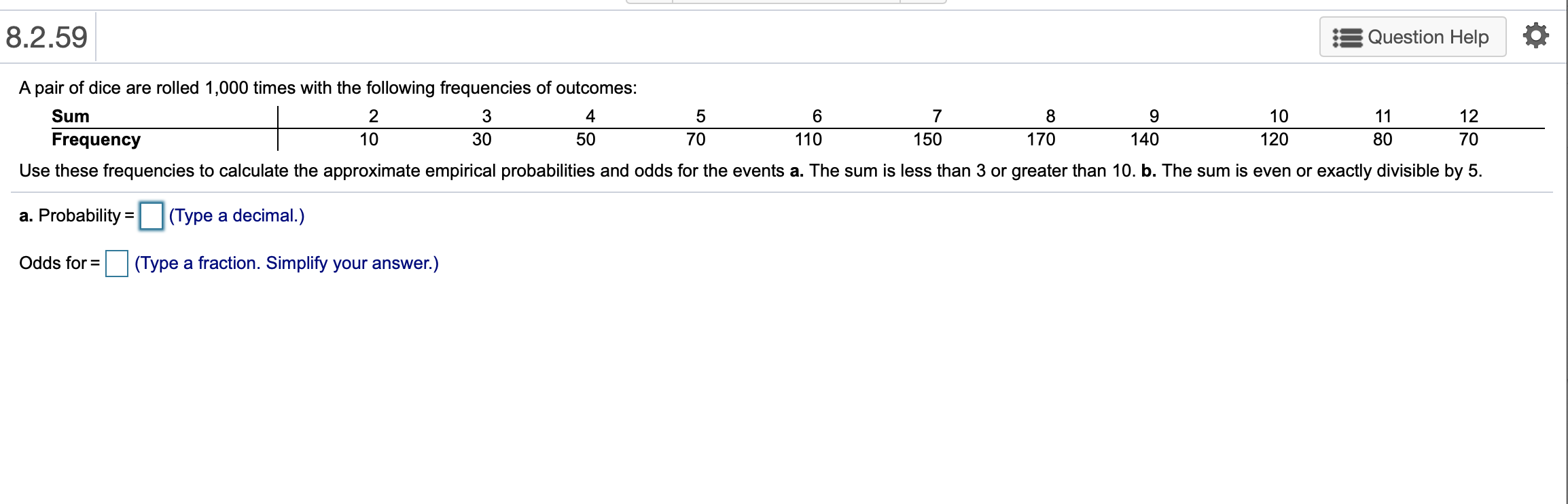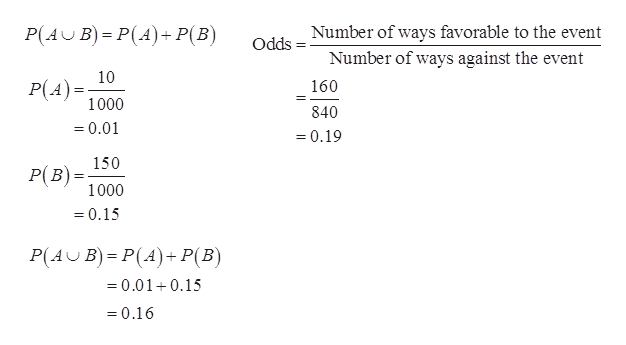# 8.2.59Question HelpA pair of dice are rolled 1,000 times with the following frequencies of outcomes:Sum2345789101112Frequency103050701101501701401208070Use these frequencies to calculate the approximate empirical probabilities and odds for the events a. The sum is less than 3 or greater than 10. b. The sum is even or exactly divisible by 5.a. Probability(Type a decimal.)Odds for(Type a fraction. Simplify your answer.)

Question
119 viewshelp_outlineImage Transcriptionclose8.2.59 Question Help A pair of dice are rolled 1,000 times with the following frequencies of outcomes: Sum 2 3 4 5 7 8 9 10 11 12 Frequency 10 30 50 70 110 150 170 140 120 80 70 Use these frequencies to calculate the approximate empirical probabilities and odds for the events a. The sum is less than 3 or greater than 10. b. The sum is even or exactly divisible by 5. a. Probability (Type a decimal.) Odds for (Type a fraction. Simplify your answer.) fullscreen
check_circle

Step 1

The formula for empirical probability is given below:

Step 2

a.

The probability of the sum less than 3 or greater than 10 is given below:

Let A is the event of getting sum is less than 3 and B is the getting sum is greater than 10.

The frequency of sum is less than 3 is 10 and the total frequency is 1000.

The probability of getting sum less than 3 is 0.01.

The probability of getting sum is greater than 10 is 0.1...help_outlineImage TranscriptioncloseP(A B) P(A) P(B) Number of ways favorable to the event Odds Number of ways against the event 10 P(A 160 1000 840 =0.01 0.19 150 PB 1000 0.15 P(A B) P(A)+ P(B) =0.010.15 =0.16 fullscreen

### Want to see the full answer?

See Solution

#### Want to see this answer and more?

Solutions are written by subject experts who are available 24/7. Questions are typically answered within 1 hour.*

See Solution
*Response times may vary by subject and question.
Tagged in

### Basic Probability